# ML Aggarwal Solutions for Class 8 Maths Chapter 9 - Direct and Inverse Variation

ML Aggarwal Solutions for Class 8 Maths Chapter 9 – Direct and Inverse Variation are provided here to help students understand the concepts clearly and score well in their exams. This chapter mainly deals with problems based on direct and inversely proportional concepts. Students who aim to secure top scores in their examinations can refer to ML Aggarwal Solutions, which is the best reference material developed by our experts, keeping in mind the latest syllabus. The solutions have been designed in a unique and simple method where any student can understand the topics clearly. Students can also learn easy tricks and short cut methods, when practised on a regular basis. The PDFs of this chapter are available here, where students can download for free from the links given below.

Chapter 9 – Direct and Inverse Variation contains three exercises and the ML Aggarwal Class 8 Solutions present in this page provides solutions to questions related to each exercise present in this chapter.

## Download the Pdf of ML Aggarwal Solutions for Class 8 Maths Chapter 9 – Direct and Inverse Variation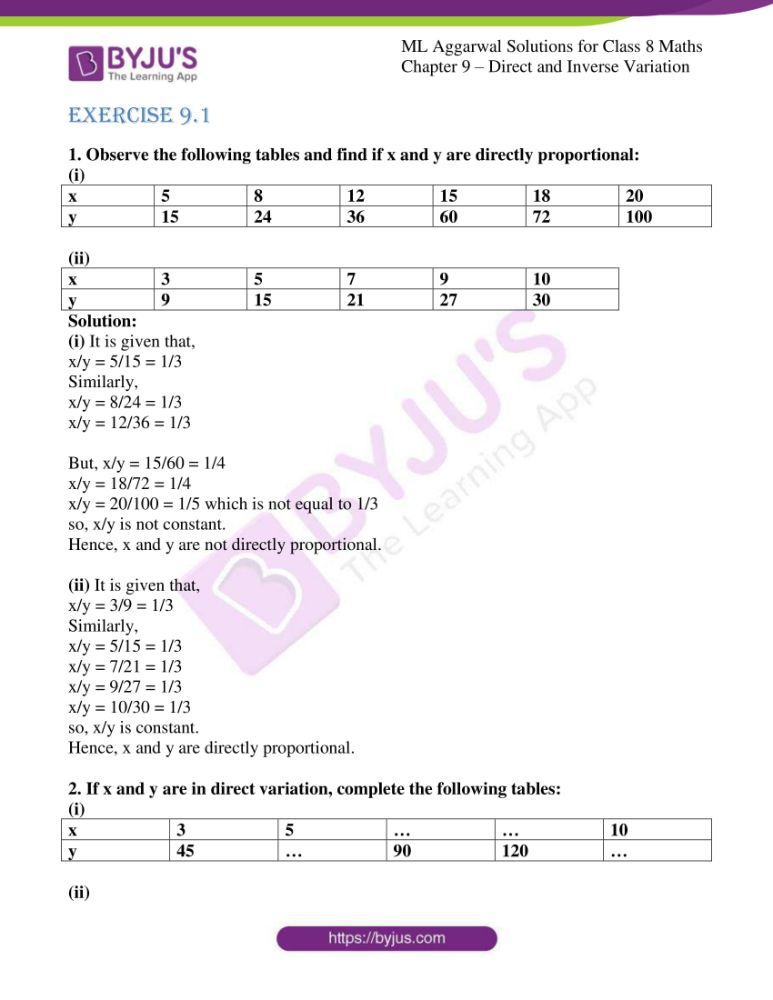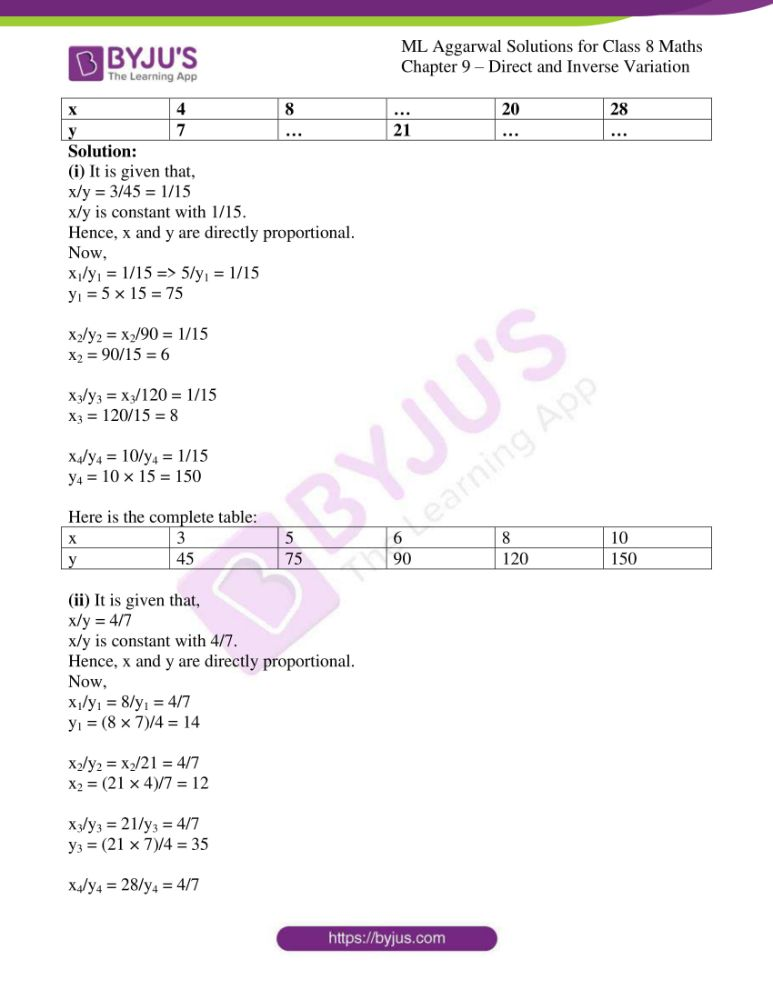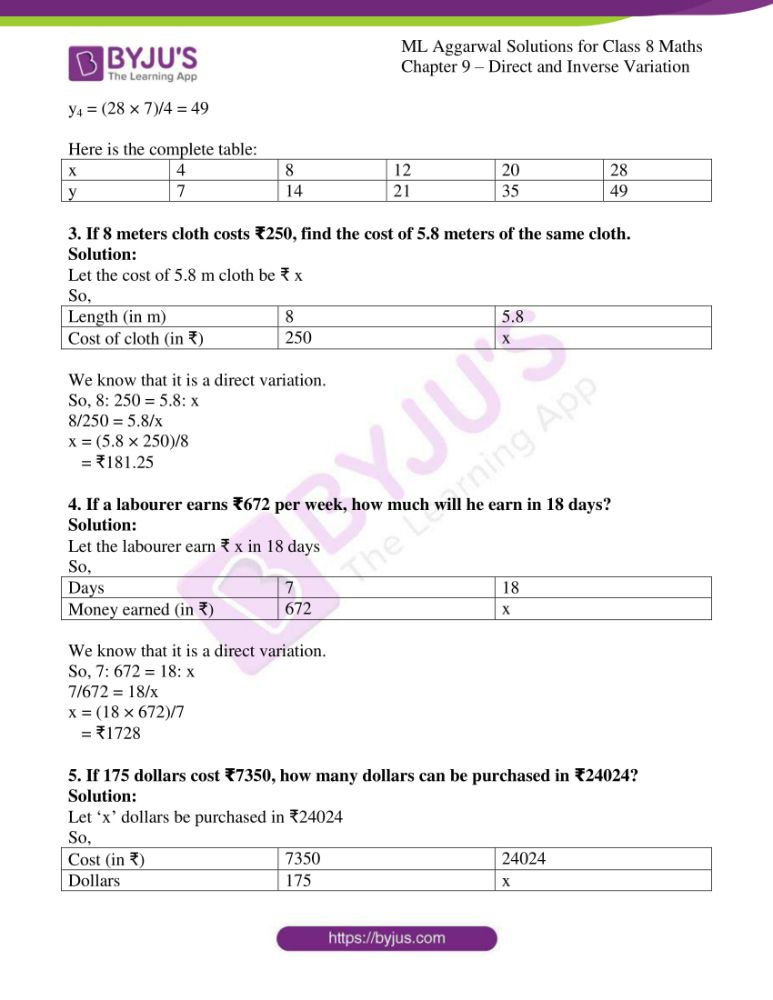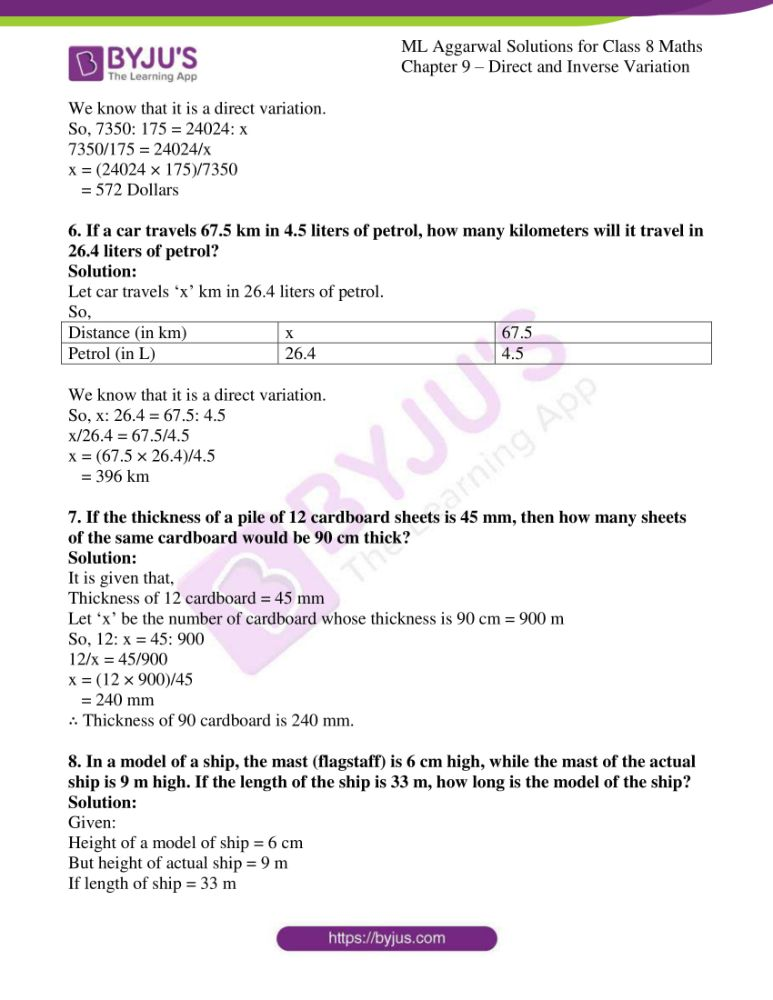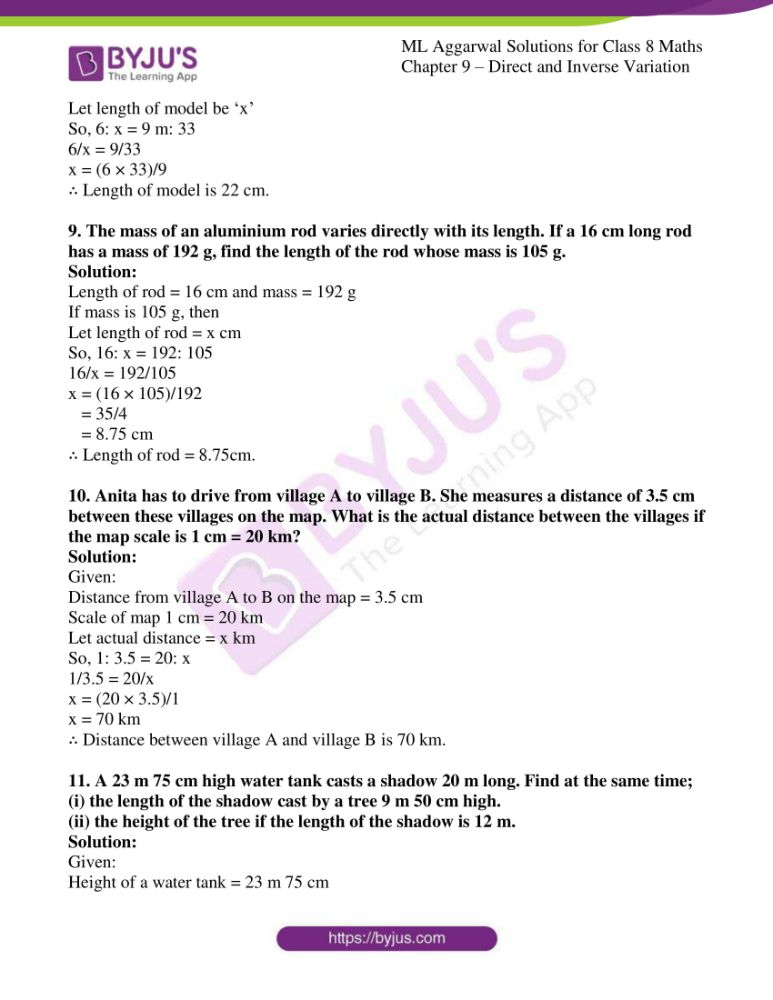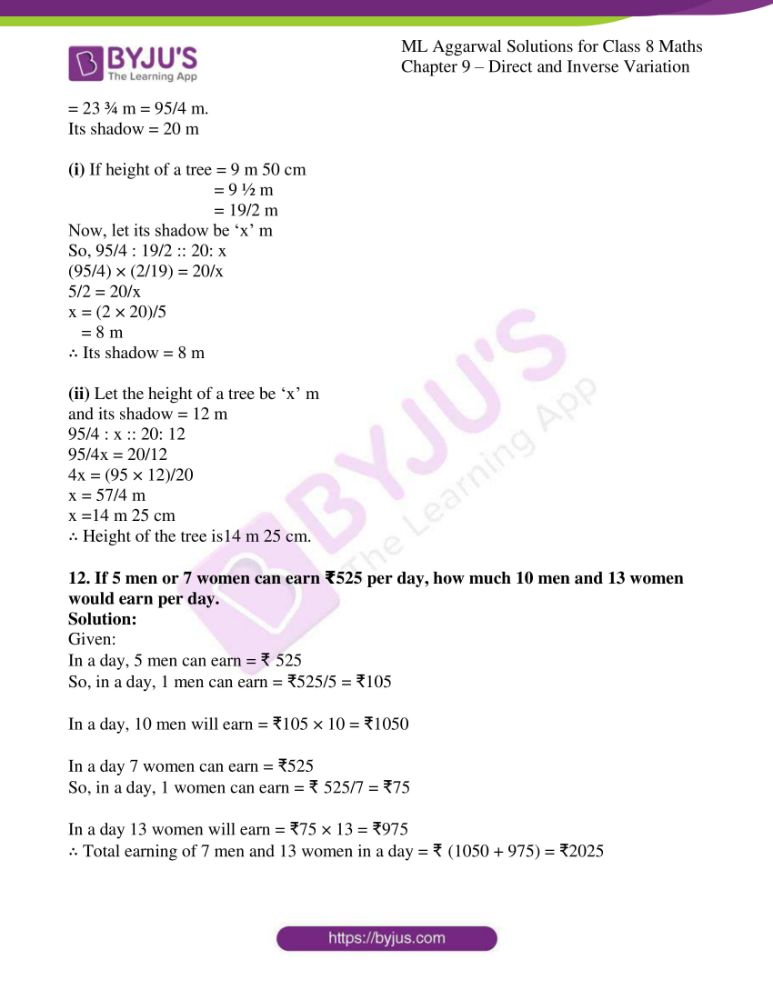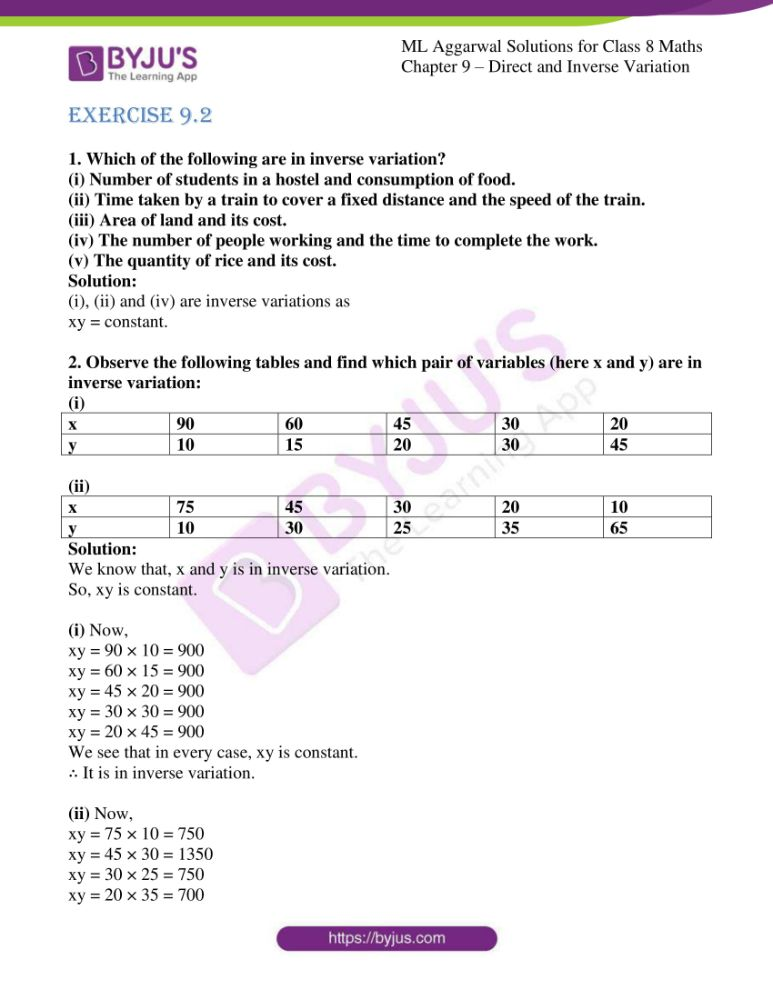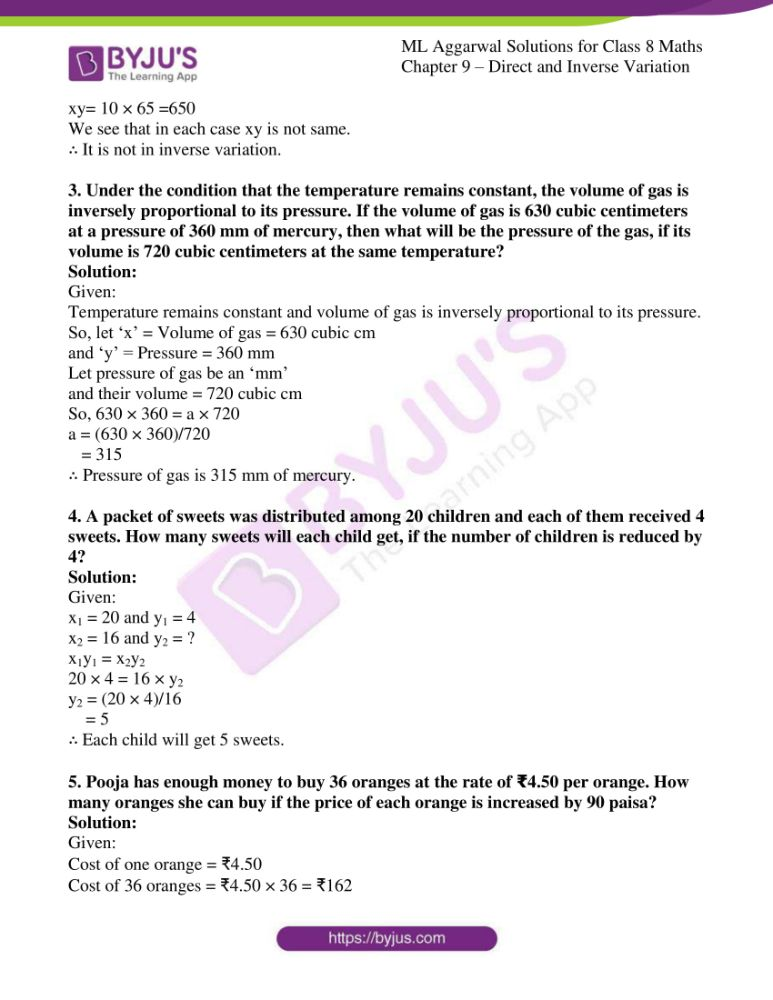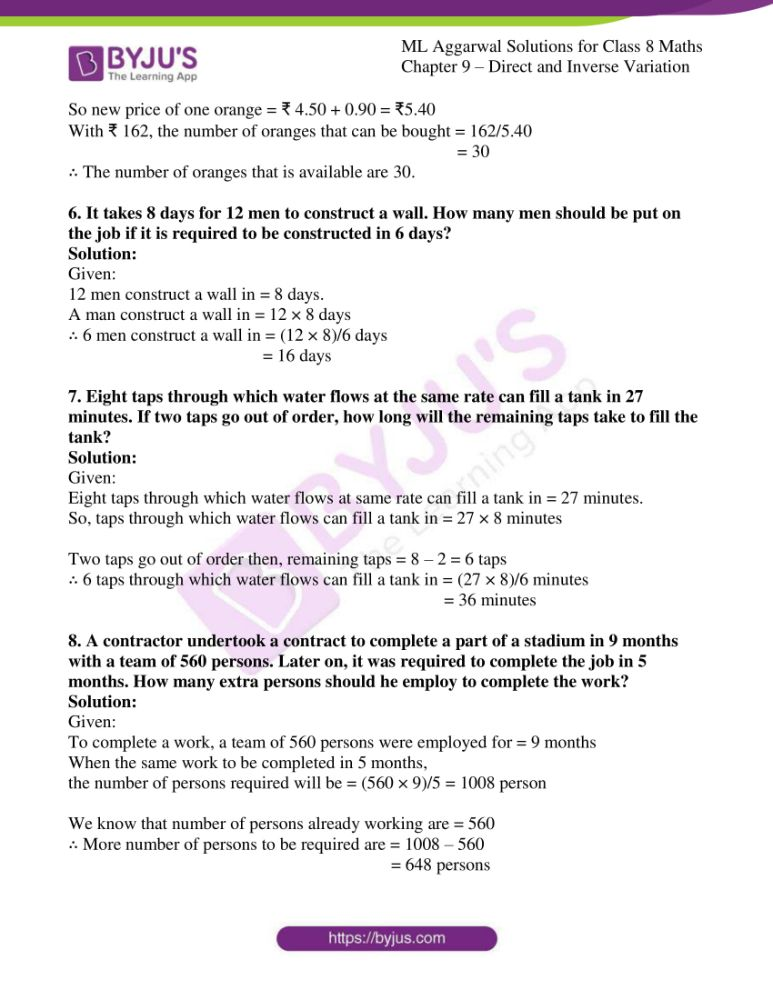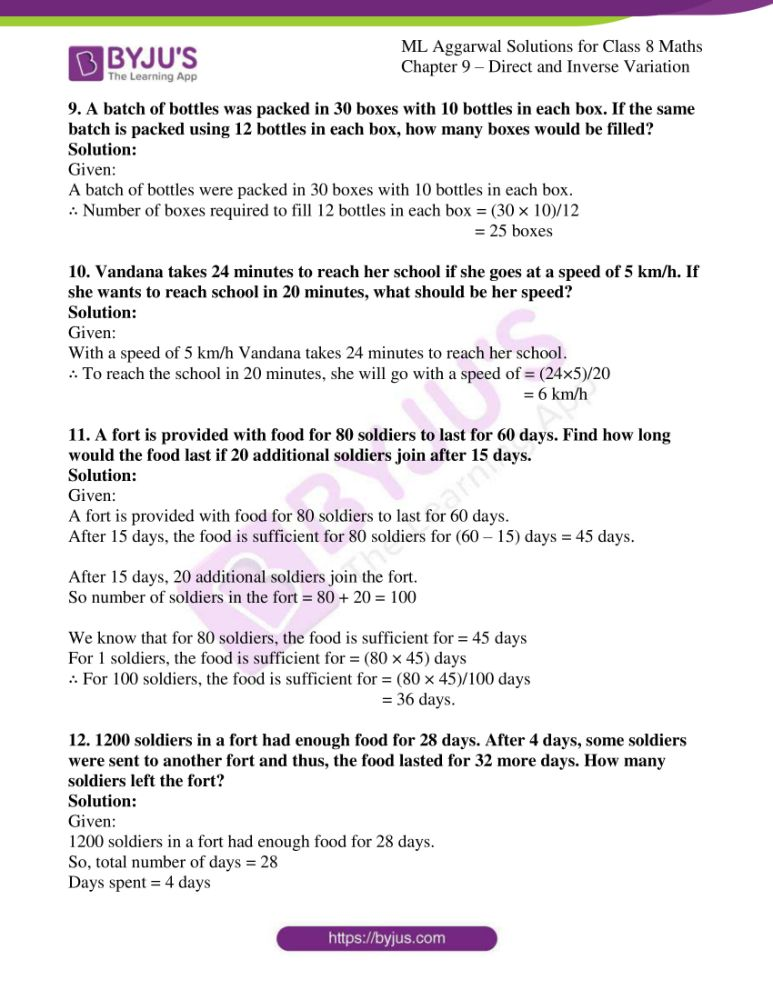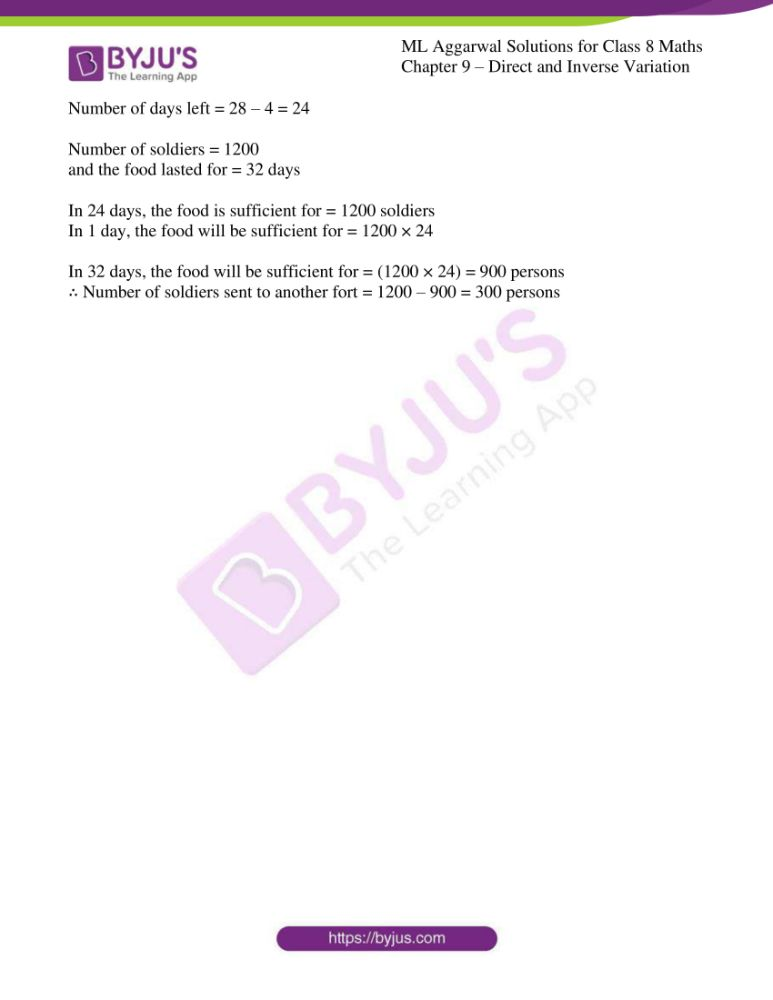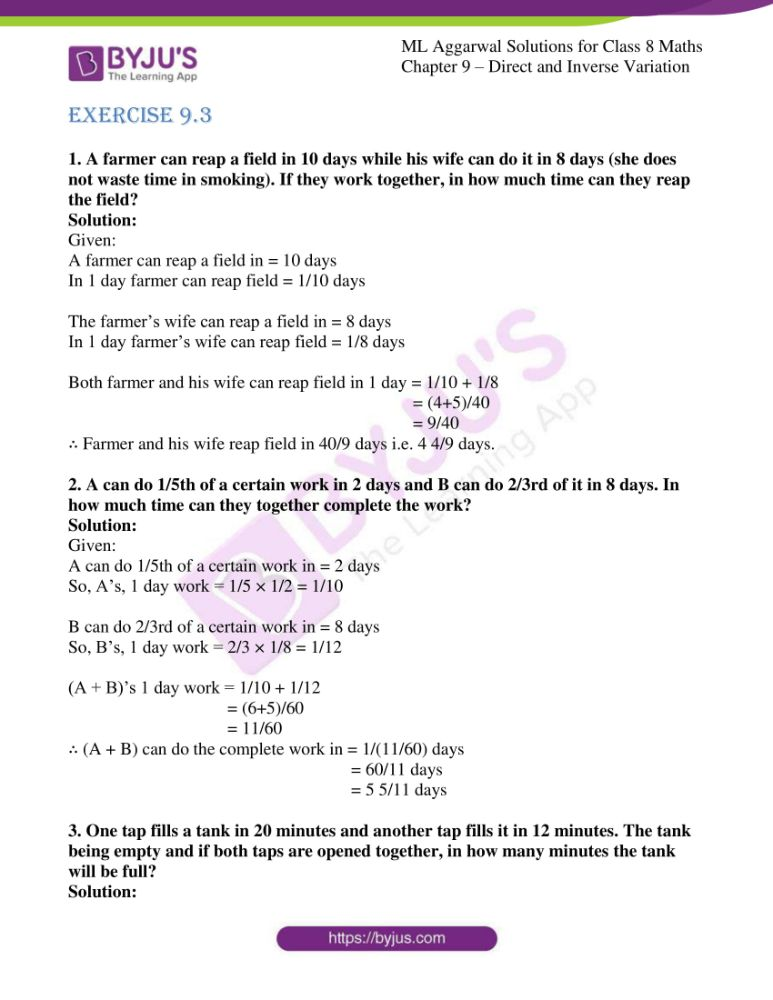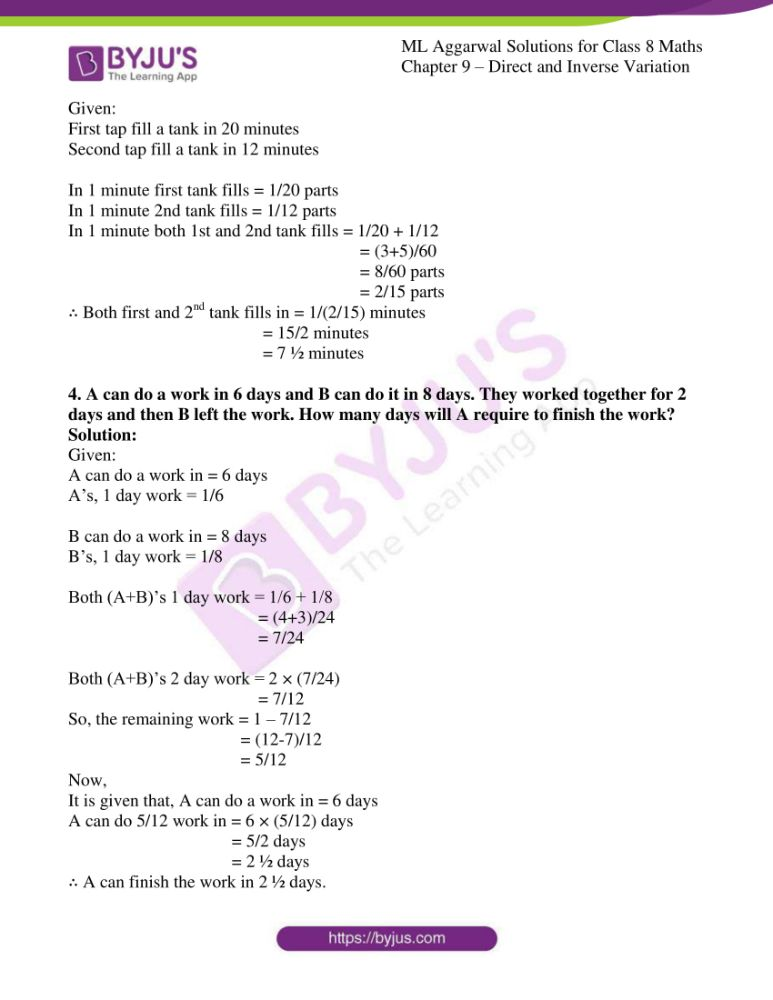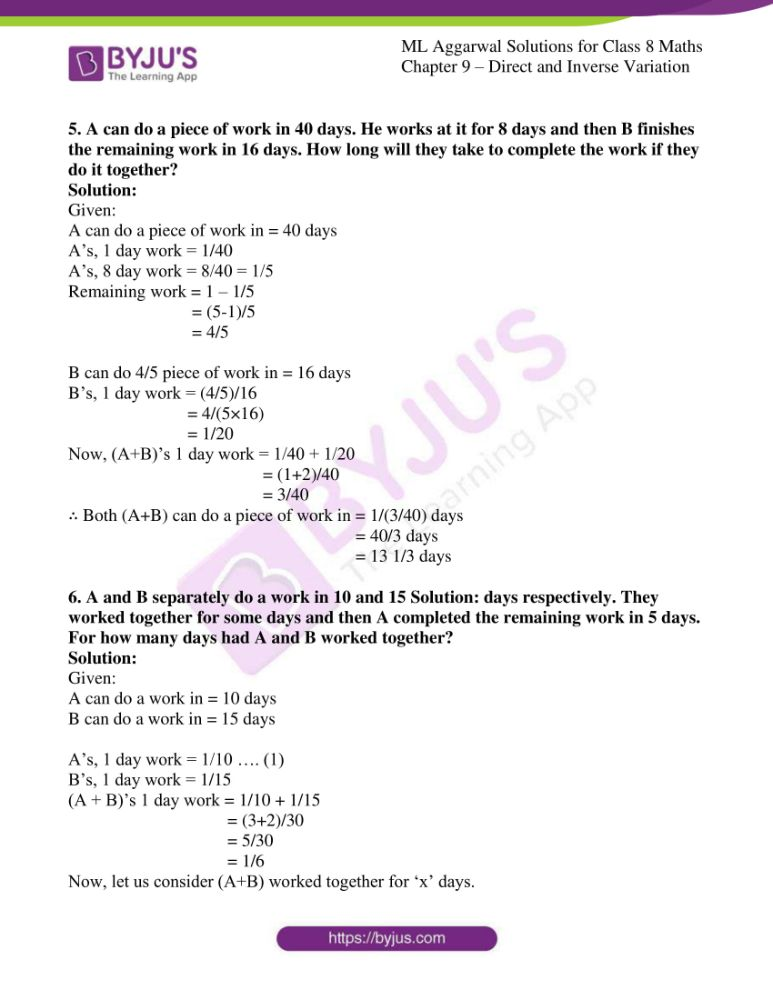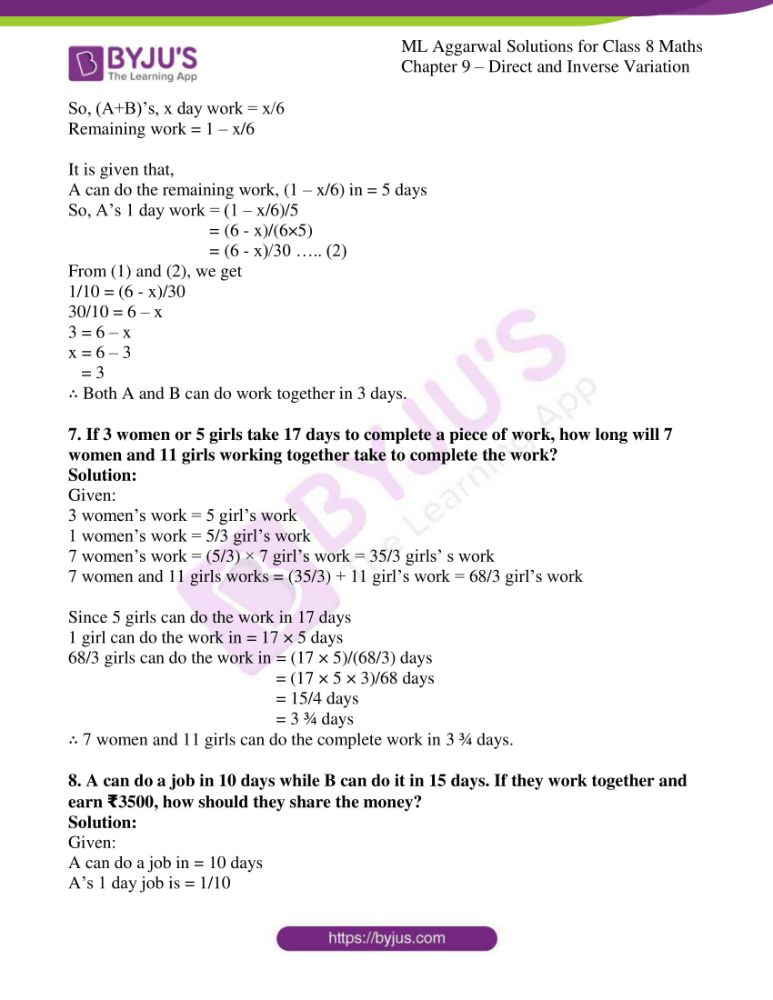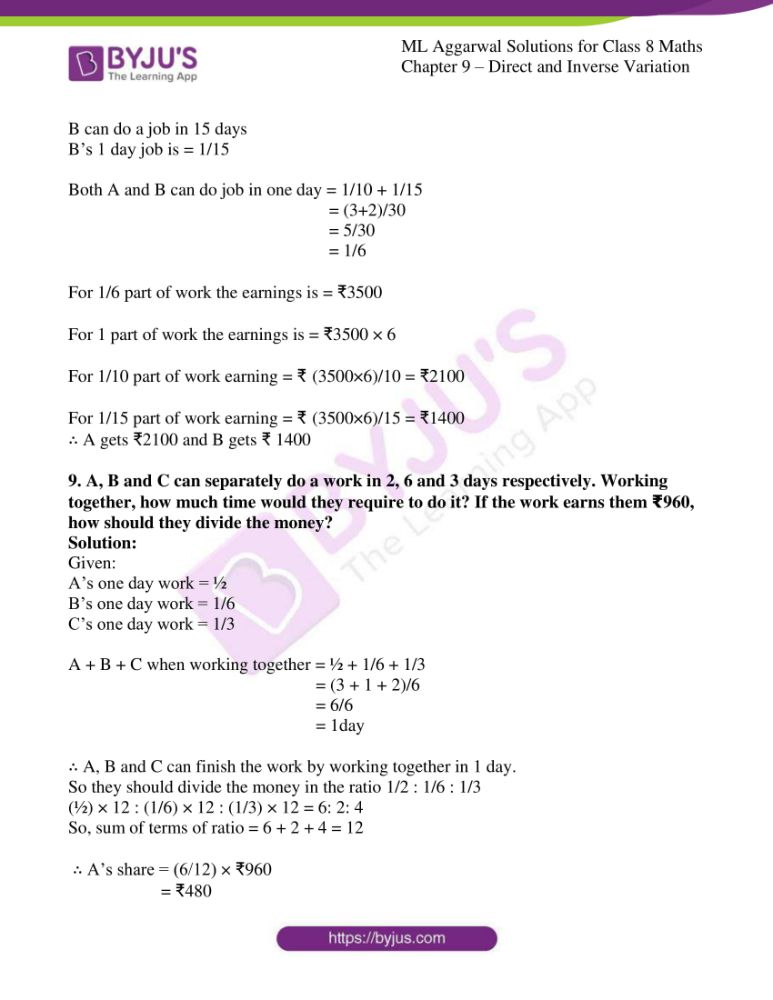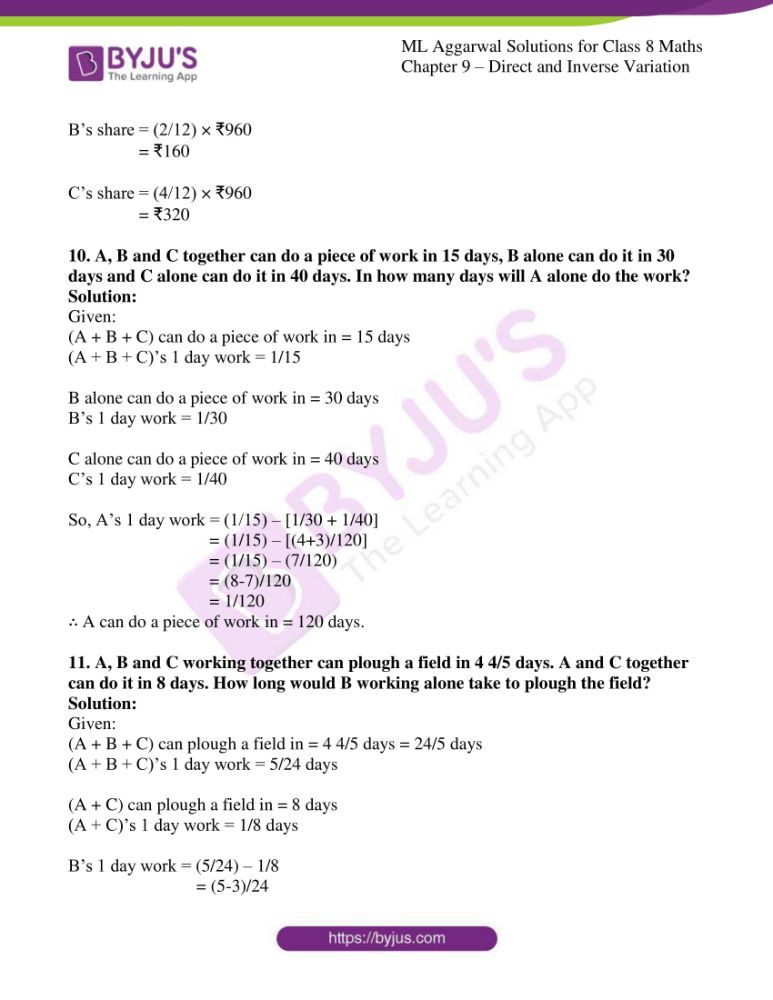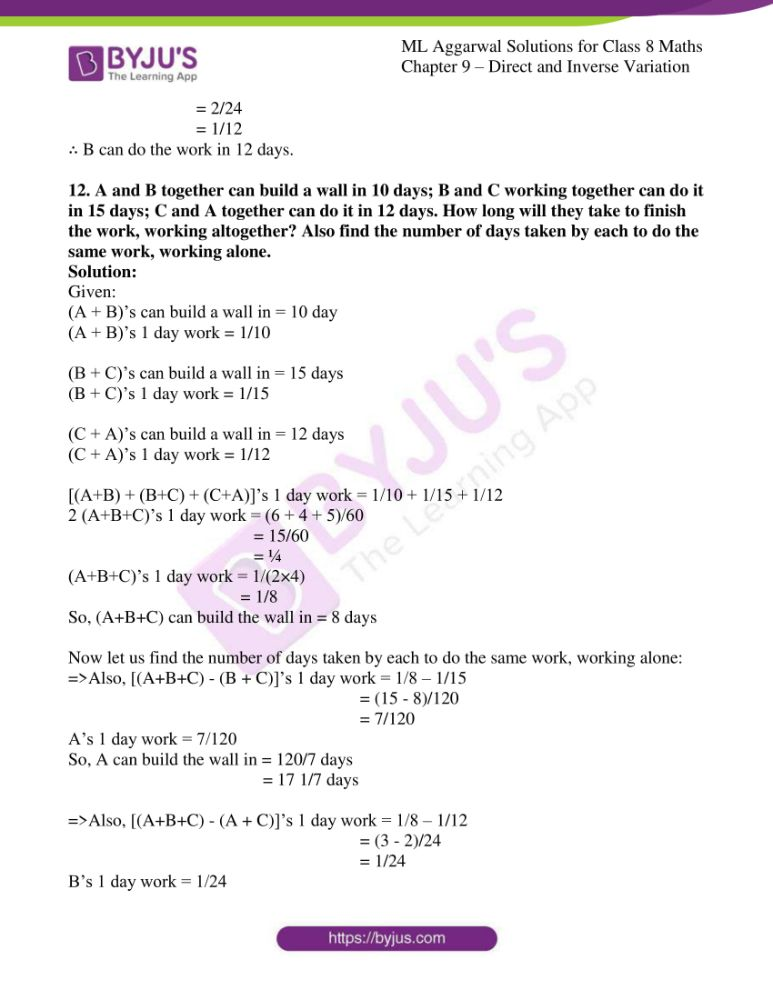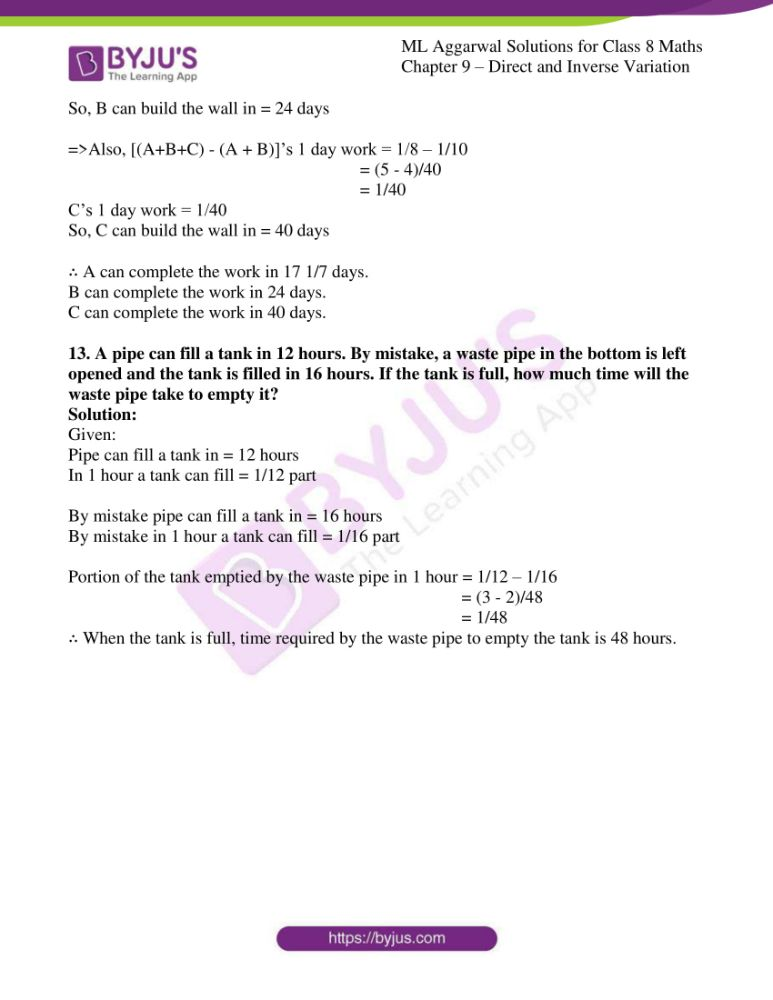### Access answers to ML Aggarwal Solutions for Class 8 Maths Chapter 9 – Direct and Inverse Variation

EXERCISE 9.1

1. Observe the following tables and find if x and y are directly proportional:
(i)

 x 5 8 12 15 18 20 y 15 24 36 60 72 100

(ii)

 x 3 5 7 9 10 y 9 15 21 27 30

Solution:

(i) It is given that,

x/y = 5/15 = 1/3

Similarly,

x/y = 8/24 = 1/3

x/y = 12/36 = 1/3

But, x/y = 15/60 = 1/4

x/y = 18/72 = 1/4

x/y = 20/100 = 1/5 which is not equal to 1/3

so, x/y is not constant.

Hence, x and y are not directly proportional.

(ii) It is given that,

x/y = 3/9 = 1/3

Similarly,

x/y = 5/15 = 1/3

x/y = 7/21 = 1/3

x/y = 9/27 = 1/3

x/y = 10/30 = 1/3

so, x/y is constant.

Hence, x and y are directly proportional.

2. If x and y are in direct variation, complete the following tables:

(i)

 x 3 5 … … 10 y 45 … 90 120 …

(ii)

 x 4 8 … 20 28 y 7 … 21 … …

Solution:

(i) It is given that,

x/y = 3/45 = 1/15

x/y is constant with 1/15.

Hence, x and y are directly proportional.

Now,

x1/y1 = 1/15 => 5/y1 = 1/15

y1 = 5 × 15 = 75

x2/y2 = x2/90 = 1/15

x2 = 90/15 = 6

x3/y3 = x3/120 = 1/15

x3 = 120/15 = 8

x4/y4 = 10/y4 = 1/15

y4 = 10 × 15 = 150

Here is the complete table:

 x 3 5 6 8 10 y 45 75 90 120 150

(ii) It is given that,

x/y = 4/7

x/y is constant with 4/7.

Hence, x and y are directly proportional.

Now,

x1/y1 = 8/y1 = 4/7

y1 = (8 × 7)/4 = 14

x2/y2 = x2/21 = 4/7

x2 = (21 × 4)/7 = 12

x3/y3 = 21/y3 = 4/7

y3 = (21 × 7)/4 = 35

x4/y4 = 28/y4 = 4/7

y4 = (28 × 7)/4 = 49

Here is the complete table:

 x 4 8 12 20 28 y 7 14 21 35 49

3. If 8 meters cloth costs ₹250, find the cost of 5.8 meters of the same cloth.
Solution:

Let the cost of 5.8 m cloth be ₹ x

So,

 Length (in m) 8 5.8 Cost of cloth (in ₹) 250 x

We know that it is a direct variation.

So, 8: 250 = 5.8: x

8/250 = 5.8/x

x = (5.8 × 250)/8

= ₹181.25

4. If a labourer earns ₹672 per week, how much will he earn in 18 days?
Solution:

Let the labourer earn ₹ x in 18 days

So,

 Days 7 18 Money earned (in ₹) 672 x

We know that it is a direct variation.

So, 7: 672 = 18: x

7/672 = 18/x

x = (18 × 672)/7

= ₹1728

5. If 175 dollars cost ₹7350, how many dollars can be purchased in ₹24024?
Solution:

Let ‘x’ dollars be purchased in ₹24024

So,

 Cost (in ₹) 7350 24024 Dollars 175 x

We know that it is a direct variation.

So, 7350: 175 = 24024: x

7350/175 = 24024/x

x = (24024 × 175)/7350

= 572 Dollars

6. If a car travels 67.5 km in 4.5 liters of petrol, how many kilometers will it travel in 26.4 liters of petrol?
Solution:

Let car travels ‘x’ km in 26.4 liters of petrol.

So,

 Distance (in km) x 67.5 Petrol (in L) 26.4 4.5

We know that it is a direct variation.

So, x: 26.4 = 67.5: 4.5

x/26.4 = 67.5/4.5

x = (67.5 × 26.4)/4.5

= 396 km

7. If the thickness of a pile of 12 cardboard sheets is 45 mm, then how many sheets of the same cardboard would be 90 cm thick?
Solution:

It is given that,

Thickness of 12 cardboard = 45 mm

Let ‘x’ be the number of cardboard whose thickness is 90 cm = 900 m

So, 12: x = 45: 900

12/x = 45/900

x = (12 × 900)/45

= 240 mm

∴ Thickness of 90 cardboard is 240 mm.

8. In a model of a ship, the mast (flagstaff) is 6 cm high, while the mast of the actual ship is 9 m high. If the length of the ship is 33 m, how long is the model of the ship?
Solution:

Given:

Height of a model of ship = 6 cm

But height of actual ship = 9 m

If length of ship = 33 m

Let length of model be ‘x’

So, 6: x = 9 m: 33

6/x = 9/33

x = (6 × 33)/9

∴ Length of model is 22 cm.

9. The mass of an aluminium rod varies directly with its length. If a 16 cm long rod has a mass of 192 g, find the length of the rod whose mass is 105 g.
Solution:

Length of rod = 16 cm and mass = 192 g

If mass is 105 g, then

Let length of rod = x cm

So, 16: x = 192: 105

16/x = 192/105

x = (16 × 105)/192

= 35/4

= 8.75 cm

∴ Length of rod = 8.75cm.

10. Anita has to drive from village A to village B. She measures a distance of 3.5 cm between these villages on the map. What is the actual distance between the villages if the map scale is 1 cm = 20 km?
Solution:

Given:

Distance from village A to B on the map = 3.5 cm

Scale of map 1 cm = 20 km

Let actual distance = x km

So, 1: 3.5 = 20: x

1/3.5 = 20/x

x = (20 × 3.5)/1

x = 70 km

∴ Distance between village A and village B is 70 km.

11. A 23 m 75 cm high water tank casts a shadow 20 m long. Find at the same time;
(i) the length of the shadow cast by a tree 9 m 50 cm high.
(ii) the height of the tree if the length of the shadow is 12 m.
Solution:

Given:

Height of a water tank = 23 m 75 cm

= 23 ¾ m = 95/4 m.

(i) If height of a tree = 9 m 50 cm

= 9 ½ m

= 19/2 m

Now, let its shadow be ‘x’ m

So, 95/4 : 19/2 :: 20: x

(95/4) × (2/19) = 20/x

5/2 = 20/x

x = (2 × 20)/5

= 8 m

∴ Its shadow = 8 m

(ii) Let the height of a tree be ‘x’ m

and its shadow = 12 m

95/4 : x :: 20: 12

95/4x = 20/12

4x = (95 × 12)/20

x = 57/4 m

x =14 m 25 cm

∴ Height of the tree is14 m 25 cm.

12. If 5 men or 7 women can earn ₹525 per day, how much 10 men and 13 women would earn per day.
Solution:

Given:

In a day, 5 men can earn = ₹ 525

So, in a day, 1 men can earn = ₹525/5 = ₹105

In a day, 10 men will earn = ₹105 × 10 = ₹1050

In a day 7 women can earn = ₹525

So, in a day, 1 women can earn = ₹ 525/7 = ₹75

In a day 13 women will earn = ₹75 × 13 = ₹975

∴ Total earning of 7 men and 13 women in a day = ₹ (1050 + 975) = ₹2025

EXERCISE 9.2

1. Which of the following are in inverse variation?
(i) Number of students in a hostel and consumption of food.
(ii) Time taken by a train to cover a fixed distance and the speed of the train.
(iii) Area of land and its cost.
(iv) The number of people working and the time to complete the work.
(v) The quantity of rice and its cost.
Solution:

(i), (ii) and (iv) are inverse variations as

xy = constant.

2. Observe the following tables and find which pair of variables (here x and y) are in inverse variation:
(i)

 x 90 60 45 30 20 y 10 15 20 30 45

(ii)

 x 75 45 30 20 10 y 10 30 25 35 65

Solution:

We know that, x and y is in inverse variation.

So, xy is constant.

(i) Now,

xy = 90 × 10 = 900

xy = 60 × 15 = 900

xy = 45 × 20 = 900

xy = 30 × 30 = 900

xy = 20 × 45 = 900

We see that in every case, xy is constant.

∴ It is in inverse variation.

(ii) Now,

xy = 75 × 10 = 750

xy = 45 × 30 = 1350

xy = 30 × 25 = 750

xy = 20 × 35 = 700

xy= 10 × 65 =650

We see that in each case xy is not same.

∴ It is not in inverse variation.

3. Under the condition that the temperature remains constant, the volume of gas is inversely proportional to its pressure. If the volume of gas is 630 cubic centimeters at a pressure of 360 mm of mercury, then what will be the pressure of the gas, if its volume is 720 cubic centimeters at the same temperature?
Solution:

Given:

Temperature remains constant and volume of gas is inversely proportional to its pressure.

So, let ‘x’ = Volume of gas = 630 cubic cm

and ‘y’ = Pressure = 360 mm

Let pressure of gas be an ‘mm’

and their volume = 720 cubic cm

So, 630 × 360 = a × 720

a = (630 × 360)/720

= 315

∴ Pressure of gas is 315 mm of mercury.

4. A packet of sweets was distributed among 20 children and each of them received 4 sweets. How many sweets will each child get, if the number of children is reduced by 4?
Solution:

Given:

x1 = 20 and y1 = 4

x2 = 16 and y2 = ?

x1y1 = x2y2

20 × 4 = 16 × y2

y2 = (20 × 4)/16

= 5

∴ Each child will get 5 sweets.

5. Pooja has enough money to buy 36 oranges at the rate of ₹4.50 per orange. How many oranges she can buy if the price of each orange is increased by 90 paisa?
Solution:

Given:

Cost of one orange = ₹4.50

Cost of 36 oranges = ₹4.50 × 36 = ₹162

So new price of one orange = ₹ 4.50 + 0.90 = ₹5.40

With ₹ 162, the number of oranges that can be bought = 162/5.40

= 30

∴ The number of oranges that is available are 30.

6. It takes 8 days for 12 men to construct a wall. How many men should be put on the job if it is required to be constructed in 6 days?
Solution:

Given:

12 men construct a wall in = 8 days.

A man construct a wall in = 12 × 8 days

∴ 6 men construct a wall in = (12 × 8)/6 days

= 16 days

7. Eight taps through which water flows at the same rate can fill a tank in 27 minutes. If two taps go out of order, how long will the remaining taps take to fill the tank?
Solution:

Given:

Eight taps through which water flows at same rate can fill a tank in = 27 minutes.

So, taps through which water flows can fill a tank in = 27 × 8 minutes

Two taps go out of order then, remaining taps = 8 – 2 = 6 taps

∴ 6 taps through which water flows can fill a tank in = (27 × 8)/6 minutes

= 36 minutes

8. A contractor undertook a contract to complete a part of a stadium in 9 months with a team of 560 persons. Later on, it was required to complete the job in 5 months. How many extra persons should he employ to complete the work?
Solution:

Given:

To complete a work, a team of 560 persons were employed for = 9 months

When the same work to be completed in 5 months,

the number of persons required will be = (560 × 9)/5 = 1008 person

We know that number of persons already working are = 560

∴ More number of persons to be required are = 1008 – 560

= 648 persons

9. A batch of bottles was packed in 30 boxes with 10 bottles in each box. If the same batch is packed using 12 bottles in each box, how many boxes would be filled?
Solution:

Given:

A batch of bottles were packed in 30 boxes with 10 bottles in each box.

∴ Number of boxes required to fill 12 bottles in each box = (30 × 10)/12

= 25 boxes

10. Vandana takes 24 minutes to reach her school if she goes at a speed of 5 km/h. If she wants to reach school in 20 minutes, what should be her speed?
Solution:

Given:

With a speed of 5 km/h Vandana takes 24 minutes to reach her school.

∴ To reach the school in 20 minutes, she will go with a speed of = (24×5)/20

= 6 km/h

11. A fort is provided with food for 80 soldiers to last for 60 days. Find how long would the food last if 20 additional soldiers join after 15 days.
Solution:

Given:

A fort is provided with food for 80 soldiers to last for 60 days.

After 15 days, the food is sufficient for 80 soldiers for (60 – 15) days = 45 days.

After 15 days, 20 additional soldiers join the fort.

So number of soldiers in the fort = 80 + 20 = 100

We know that for 80 soldiers, the food is sufficient for = 45 days

For 1 soldiers, the food is sufficient for = (80 × 45) days

∴ For 100 soldiers, the food is sufficient for = (80 × 45)/100 days

= 36 days.

12. 1200 soldiers in a fort had enough food for 28 days. After 4 days, some soldiers were sent to another fort and thus, the food lasted for 32 more days. How many soldiers left the fort?
Solution:

Given:

1200 soldiers in a fort had enough food for 28 days.

So, total number of days = 28

Days spent = 4 days

Number of days left = 28 – 4 = 24

Number of soldiers = 1200

and the food lasted for = 32 days

In 24 days, the food is sufficient for = 1200 soldiers

In 1 day, the food will be sufficient for = 1200 × 24

In 32 days, the food will be sufficient for = (1200 × 24) = 900 persons

∴ Number of soldiers sent to another fort = 1200 – 900 = 300 persons

EXERCISE 9.3

1. A farmer can reap a field in 10 days while his wife can do it in 8 days (she does not waste time in smoking). If they work together, in how much time can they reap the field?
Solution:

Given:

A farmer can reap a field in = 10 days

In 1 day farmer can reap field = 1/10 days

The farmer’s wife can reap a field in = 8 days

In 1 day farmer’s wife can reap field = 1/8 days

Both farmer and his wife can reap field in 1 day = 1/10 + 1/8

= (4+5)/40

= 9/40

∴ Farmer and his wife reap field in 40/9 days i.e. 4 4/9 days.

2. A can do 1/5th of a certain work in 2 days and B can do 2/3rd of it in 8 days. In how much time can they together complete the work?
Solution:

Given:

A can do 1/5th of a certain work in = 2 days

So, A’s, 1 day work = 1/5 × 1/2 = 1/10

B can do 2/3rd of a certain work in = 8 days

So, B’s, 1 day work = 2/3 × 1/8 = 1/12

(A + B)’s 1 day work = 1/10 + 1/12

= (6+5)/60

= 11/60

∴ (A + B) can do the complete work in = 1/(11/60) days

= 60/11 days

= 5 5/11 days

3. One tap fills a tank in 20 minutes and another tap fills it in 12 minutes. The tank being empty and if both taps are opened together, in how many minutes the tank will be full?
Solution:

Given:

First tap fill a tank in 20 minutes

Second tap fill a tank in 12 minutes

In 1 minute first tank fills = 1/20 parts

In 1 minute 2nd tank fills = 1/12 parts

In 1 minute both 1st and 2nd tank fills = 1/20 + 1/12

= (3+5)/60

= 8/60 parts

= 2/15 parts

∴ Both first and 2nd tank fills in = 1/(2/15) minutes

= 15/2 minutes

= 7 ½ minutes

4. A can do a work in 6 days and B can do it in 8 days. They worked together for 2 days and then B left the work. How many days will A require to finish the work?
Solution:

Given:

A can do a work in = 6 days

A’s, 1 day work = 1/6

B can do a work in = 8 days

B’s, 1 day work = 1/8

Both (A+B)’s 1 day work = 1/6 + 1/8

= (4+3)/24

= 7/24

Both (A+B)’s 2 day work = 2 × (7/24)

= 7/12

So, the remaining work = 1 – 7/12

= (12-7)/12

= 5/12

Now,

It is given that, A can do a work in = 6 days

A can do 5/12 work in = 6 × (5/12) days

= 5/2 days

= 2 ½ days

∴ A can finish the work in 2 ½ days.

5. A can do a piece of work in 40 days. He works at it for 8 days and then B finishes the remaining work in 16 days. How long will they take to complete the work if they do it together?
Solution:

Given:

A can do a piece of work in = 40 days

A’s, 1 day work = 1/40

A’s, 8 day work = 8/40 = 1/5

Remaining work = 1 – 1/5

= (5-1)/5

= 4/5

B can do 4/5 piece of work in = 16 days

B’s, 1 day work = (4/5)/16

= 4/(5×16)

= 1/20

Now, (A+B)’s 1 day work = 1/40 + 1/20

= (1+2)/40

= 3/40

∴ Both (A+B) can do a piece of work in = 1/(3/40) days

= 40/3 days

= 13 1/3 days

6. A and B separately do a work in 10 and 15 Solution: days respectively. They worked together for some days and then A completed the remaining work in 5 days. For how many days had A and B worked together?
Solution:

Given:

A can do a work in = 10 days

B can do a work in = 15 days

A’s, 1 day work = 1/10 …. (1)

B’s, 1 day work = 1/15

(A + B)’s 1 day work = 1/10 + 1/15

= (3+2)/30

= 5/30

= 1/6

Now, let us consider (A+B) worked together for ‘x’ days.

So, (A+B)’s, x day work = x/6

Remaining work = 1 – x/6

It is given that,

A can do the remaining work, (1 – x/6) in = 5 days

So, A’s 1 day work = (1 – x/6)/5

= (6 – x)/(6×5)

= (6 – x)/30 ….. (2)

From (1) and (2), we get

1/10 = (6 – x)/30

30/10 = 6 – x

3 = 6 – x

x = 6 – 3

= 3

∴ Both A and B can do work together in 3 days.

7. If 3 women or 5 girls take 17 days to complete a piece of work, how long will 7 women and 11 girls working together take to complete the work?
Solution:

Given:

3 women’s work = 5 girl’s work

1 women’s work = 5/3 girl’s work

7 women’s work = (5/3) × 7 girl’s work = 35/3 girls’ s work

7 women and 11 girls works = (35/3) + 11 girl’s work = 68/3 girl’s work

Since 5 girls can do the work in 17 days

1 girl can do the work in = 17 × 5 days

68/3 girls can do the work in = (17 × 5)/(68/3) days

= (17 × 5 × 3)/68 days

= 15/4 days

= 3 ¾ days

∴ 7 women and 11 girls can do the complete work in 3 ¾ days.

8. A can do a job in 10 days while B can do it in 15 days. If they work together and earn ₹3500, how should they share the money?
Solution:

Given:

A can do a job in = 10 days

A’s 1 day job is = 1/10

B can do a job in 15 days

B’s 1 day job is = 1/15

Both A and B can do job in one day = 1/10 + 1/15

= (3+2)/30

= 5/30

= 1/6

For 1/6 part of work the earnings is = ₹3500

For 1 part of work the earnings is = ₹3500 × 6

For 1/10 part of work earning = ₹ (3500×6)/10 = ₹2100

For 1/15 part of work earning = ₹ (3500×6)/15 = ₹1400

∴ A gets ₹2100 and B gets ₹ 1400

9. A, B and C can separately do a work in 2, 6 and 3 days respectively. Working together, how much time would they require to do it? If the work earns them ₹960, how should they divide the money?
Solution:

Given:

A’s one day work = ½

B’s one day work = 1/6

C’s one day work = 1/3

A + B + C when working together = ½ + 1/6 + 1/3

= (3 + 1 + 2)/6

= 6/6

= 1day

∴ A, B and C can finish the work by working together in 1 day.

So they should divide the money in the ratio 1/2 : 1/6 : 1/3

(½) × 12 : (1/6) × 12 : (1/3) × 12 = 6: 2: 4

So, sum of terms of ratio = 6 + 2 + 4 = 12

∴ A’s share = (6/12) × ₹960

= ₹480

B’s share = (2/12) × ₹960

= ₹160

C’s share = (4/12) × ₹960

= ₹320

10. A, B and C together can do a piece of work in 15 days, B alone can do it in 30 days and C alone can do it in 40 days. In how many days will A alone do the work?
Solution:

Given:

(A + B + C) can do a piece of work in = 15 days

(A + B + C)’s 1 day work = 1/15

B alone can do a piece of work in = 30 days

B’s 1 day work = 1/30

C alone can do a piece of work in = 40 days

C’s 1 day work = 1/40

So, A’s 1 day work = (1/15) – [1/30 + 1/40]

= (1/15) – [(4+3)/120]

= (1/15) – (7/120)

= (8-7)/120

= 1/120

∴ A can do a piece of work in = 120 days.

11. A, B and C working together can plough a field in 4 4/5 days. A and C together can do it in 8 days. How long would B working alone take to plough the field?
Solution:

Given:

(A + B + C) can plough a field in = 4 4/5 days = 24/5 days

(A + B + C)’s 1 day work = 5/24 days

(A + C) can plough a field in = 8 days

(A + C)’s 1 day work = 1/8 days

B’s 1 day work = (5/24) – 1/8

= (5-3)/24

= 2/24

= 1/12

∴ B can do the work in 12 days.

12. A and B together can build a wall in 10 days; B and C working together can do it in 15 days; C and A together can do it in 12 days. How long will they take to finish the work, working altogether? Also find the number of days taken by each to do the same work, working alone.
Solution:

Given:

(A + B)’s can build a wall in = 10 day

(A + B)’s 1 day work = 1/10

(B + C)’s can build a wall in = 15 days

(B + C)’s 1 day work = 1/15

(C + A)’s can build a wall in = 12 days

(C + A)’s 1 day work = 1/12

[(A+B) + (B+C) + (C+A)]’s 1 day work = 1/10 + 1/15 + 1/12

2 (A+B+C)’s 1 day work = (6 + 4 + 5)/60

= 15/60

= ¼

(A+B+C)’s 1 day work = 1/(2×4)

= 1/8

So, (A+B+C) can build the wall in = 8 days

Now let us find the number of days taken by each to do the same work, working alone:

=>Also, [(A+B+C) – (B + C)]’s 1 day work = 1/8 – 1/15

= (15 – 8)/120

= 7/120

A’s 1 day work = 7/120

So, A can build the wall in = 120/7 days

= 17 1/7 days

=>Also, [(A+B+C) – (A + C)]’s 1 day work = 1/8 – 1/12

= (3 – 2)/24

= 1/24

B’s 1 day work = 1/24

So, B can build the wall in = 24 days

=>Also, [(A+B+C) – (A + B)]’s 1 day work = 1/8 – 1/10

= (5 – 4)/40

= 1/40

C’s 1 day work = 1/40

So, C can build the wall in = 40 days

∴ A can complete the work in 17 1/7 days.

B can complete the work in 24 days.

C can complete the work in 40 days.

13. A pipe can fill a tank in 12 hours. By mistake, a waste pipe in the bottom is left opened and the tank is filled in 16 hours. If the tank is full, how much time will the waste pipe take to empty it?
Solution:

Given:

Pipe can fill a tank in = 12 hours

In 1 hour a tank can fill = 1/12 part

By mistake pipe can fill a tank in = 16 hours

By mistake in 1 hour a tank can fill = 1/16 part

Portion of the tank emptied by the waste pipe in 1 hour = 1/12 – 1/16

= (3 – 2)/48

= 1/48

∴ When the tank is full, time required by the waste pipe to empty the tank is 48 hours.